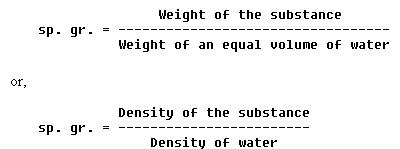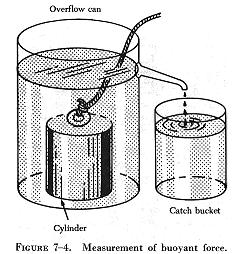FLUIDS FLUIDS

General

Because both liquids and gases flow freely, they are called fluids, from the Latin word "fluidus," meaning to flow. A fluid is defined as a substance which changes its shape easily and takes the shape of its container. This applies to both liquids and gases. The characteristics of liquids and gases may be grouped under similarities and differences. Similar characteristics are as follows:

1. Each has no definite shape but conforms to the shape of the container.
2. Both readily transmit pressures.

Differential characteristics are as follows:

1. Gases fill their containers completely, but liquids may not.
2. Gases are lighter than equal volumes of liquids.
3. Gases are highly compressible, but liquids are only slightly so.

These differences are described in the appropriate areas of the following discussion concerning the properties and characteristics of fluids at rest. Also included are some of the factors which affect fluids in different situations.

Density and Specific Gravity

The density of a substance is its weight per unit volume. The unit volume selected for use in the English system of measurement is 1 cubic foot. In the metric system it is 1 cubic centimeter. Therefore, density is expressed in lbs/cu ft (pounds per cubic foot) or in g./cu cm. (grams per cubic centimeter).

To find the density of a substance, its weight and volume must be known. Its weight is then divided by its volume to find the weight per unit volume.

For example, the liquid which fills a certain container weighs 1,497.6 pounds. The container is 4 feet long, 3 feet wide, and 2 feet deep. Its volume is 24 cubic feet (4 ft x 3 ft x 2 ft). If 24 cubic feet of liquid weights 1,497.6 pounds, then 1 cubic foot weighs 1,497.6 / 24, or 62.4 pounds. Therefore, the density of the liquid is 62.4 lbs/cu ft.

This is the density of water at 4° C (centigrade) and is usually used as the standard for comparing densities of other substances. (In the metric system the density of water is 1 g./cu cm.). The standard temperature of 4° C is used when measuring the density of liquids and solids. Changes in temperature will not change the weight of a substance, but will change the volume of the substance by expansion or contraction, thus changing its weight per unit volume.

The procedure for finding density applies to all substances; however, it is necessary to consider the pressure when finding the density of gases. Temperature is more critical when measuring the density of gases than it is for other substances. The density of a gas increases in direct proportion to the pressure exerted on it. Standard conditions for the measurement of the densities of gases have been established at 0° C for temperature and a pressure of 76 cm. of mercury. (This is the average pressure of the atmosphere at sea level.) Density is computed based on these conditions for all gases.

It is often necessary to compare the density of one substance with that of another. For this purpose, a standard is needed. Water is the standard that physicists have chosen to use when comparing the densities of all liquids and solids. For gases, air is most commonly used. However, hydrogen is sometimes used as a standard for gases. In physics, the word specific implies a ratio. Thus, specific gravity is calculated by comparing the weight of a definite volume of the given substance with the weight of an equal volume of water. The terms "specific weight" or "specific density" are sometimes used to express this ratio.

The following formulas are used to find the sp. gr. (specific gravity) of liquids and solids:The same formulas are used to find the density of gases by substituting air or hydrogen for water.

Specific gravity is not expressed in units, but as pure numbers. For example, if a certain hydraulic liquid has a specific gravity of 0.8, 1 cu ft of the liquid weighs 0.8 times as much as 1 cu ft of water: 62.4 times 0.8 or 49.92 pounds.

In the metric system, 1 cu cm of a substance with a specific gravity of 0.8 weighs 1 times 0.8 or 0.8 gr (Note that in the metric system the specific gravity of a liquid or solid has the same numerical value as its density. Since air weighs 1.293 gr/L (grams per liter), the specific gravity of gases does not equal the metric densities.)

Specific gravity and density are independent of the size of the sample under consideration and depend only upon the substance of which it is made. See figure 7-2 for typical values of sp. gr. for various substances.

A device called a hydrometer is used for measuring specific gravity of liquids. This device consists of a tubular shaped glass float contained in a larger glass tube. (See figure 7-3.) The larger glass tube provides the container for the liquid. A rubber suction bulb draws the liquid up into the container. There must be enough liquid to raise the float and prevent it from touching the bottom. The float is weighted and has a vertically graduated scale. To determine specific gravity, the scale is read at the surface of the liquid in which the float is immersed. An indication of 1000 is read when the float is immersed in pure water. When immersed in a liquid of greater density, the float rises, indicating a greater specific gravity. For liquids of lesser density the float sinks, indicating a lower specific gravity.

An example of the use of the hydrometer is its use in determining the specific gravity of the electrolyte (battery liquid) in an aircraft battery. When a battery is discharged, the calibrated float immersed in the electrolyte will indicate approximately 1150. The indication of a charged battery is between 1275 and 1310.

Buoyancy

A solid body submerged in a liquid or a gas weighs less than when weighed in free space. This is because of the upward force, called buoyant force, which any fluid exerts on a body submerged in it. An object will float if this upward force of the fluid is greater than the weight of the object. Objects denser than the fluid, even though they sink readily, appear to lose a part of their weight when submerged. A person can lift a larger weight under water than he can possibly lift in the air.

 The following experiment is illustrated in figure 7-4. The overflow can is filled up to the spout with water. The heavy metal cylinder is first weighed in still air and then is weighed while completely submerged in the water. The difference between the two weights is the buoyant force of the water. As the cylinder is lowered into the overflow can, the water is caught in the catch bucket. The volume of water which overflows equals the volume of the cylinder. (The volume of irregular shaped objects can be measured by this method.) If this experiment is performed carefully, the weight of the water displaced by the metal cylinder exactly equals the buoyant force of the water.Similar experiments were performed by Archimedes (287 - 212 B.C.). As a result of his experiments, he discovered that the buoyant force which a fluid exerts upon a submerged body is equal to the weight of the fluid the body displaces. This statement is referred to as Archimedes' principle. This principle applies to all fluids, gases as well as liquids. Just as water exerts a buoyant force on submerged objects, air exerts a buoyant force on objects submerged in it.